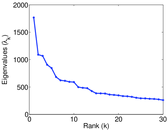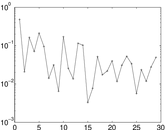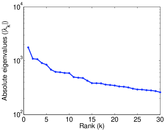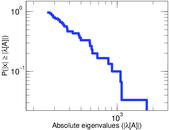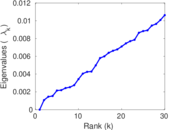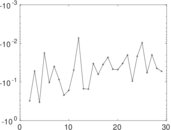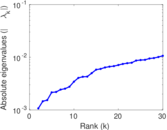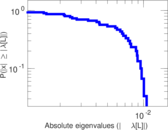# BibSonomy user–item

This is the bipartite user–publication network from BibSonomy. Each edge represents a tag assignment. The network allows multiple edge, i.e., a user may give multiple tags to a single publication. The network is complete as it is taken from the official BibSonomy dump.

 Code `Bui` Internal name `bibsonomy-2ui` Name BibSonomy user–item Data source http://www.kde.cs.uni-kassel.de/bibsonomy/dumps AvailabilityDataset is available for download Consistency checkDataset passed all tests Category Interaction network Dataset timestamp 2010 Node meaning User, publication Edge meaning Tag assignment Network formatBipartite, undirected Edge typeUnweighted, multiple edges Temporal dataEdges are annotated with timestamps

## Statistics

 Size n = 773,241 Left size n1 = 5,794 Right size n2 = 767,447 Volume m = 2,555,080 Unique edge count m̿ = 801,784 Wedge count s = 12,835,279,754 Claw count z = 394,424,715,096,067 Cross count x = 1.037 59 × 1019 Square count q = 2,794,766 4-Tour count T4 = 51,365,102,888 Maximum degree dmax = 428,436 Maximum left degree d1max = 428,436 Maximum right degree d2max = 341 Average degree d = 6.608 75 Average left degree d1 = 440.987 Average right degree d2 = 3.329 32 Fill p = 0.000 180 314 Average edge multiplicity m̃ = 3.186 74 Size of LCC N = 349,470 Diameter δ = 16 50-Percentile effective diameter δ0.5 = 3.763 18 90-Percentile effective diameter δ0.9 = 5.788 28 Median distance δM = 4 Mean distance δm = 4.698 34 Gini coefficient G = 0.730 014 Balanced inequality ratio P = 0.222 665 Left balanced inequality ratio P1 = 0.080 483 2 Right balanced inequality ratio P2 = 0.324 171 Relative edge distribution entropy Her = 0.738 449 Power law exponent γ = 29.250 6 Tail power law exponent γt = 4.691 00 Tail power law exponent with p γ3 = 4.691 00 p-value p = 0.000 00 Left tail power law exponent with p γ3,1 = 1.431 00 Left p-value p1 = 0.000 00 Right tail power law exponent with p γ3,2 = 5.281 00 Right p-value p2 = 0.000 00 Degree assortativity ρ = −0.087 479 1 Degree assortativity p-value pρ = 0.000 00 Spectral norm α = 1,767.75 Algebraic connectivity a = 0.001 069 24 Spectral separation |λ1[A] / λ2[A]| = 1.621 81 Controllability C = 761,785 Relative controllability Cr = 0.985 184

## Plots

### Fruchterman–Reingold graph drawing### Degree distribution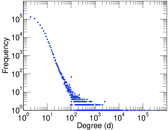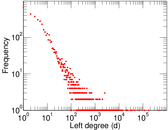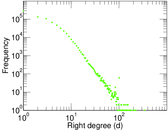### Cumulative degree distribution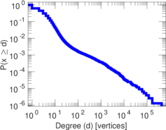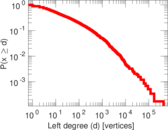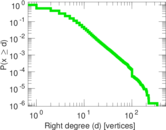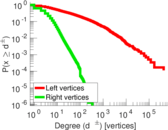### Lorenz curve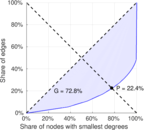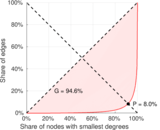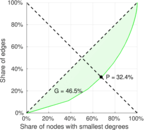### Spectral distribution of the adjacency matrix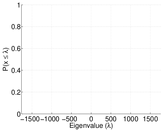### Spectral distribution of the normalized adjacency matrix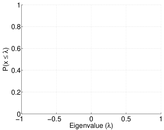### Spectral distribution of the Laplacian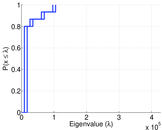### Spectral graph drawing based on the adjacency matrix### Spectral graph drawing based on the Laplacian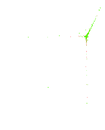### Spectral graph drawing based on the normalized adjacency matrix### Degree assortativity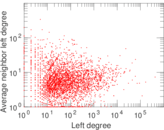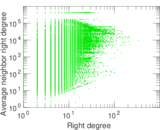### Zipf plot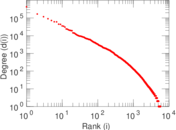### Hop distribution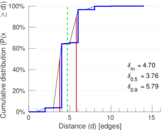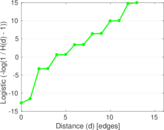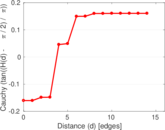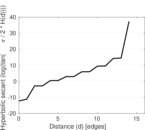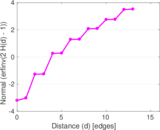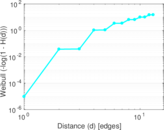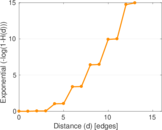### Edge weight/multiplicity distribution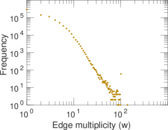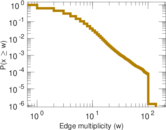### Temporal distribution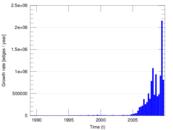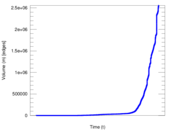### Diameter/density evolution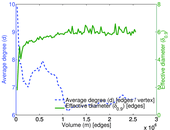### Inter-event distribution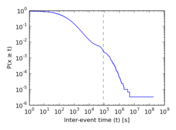### Node-level inter-event distribution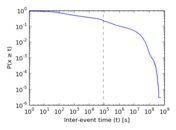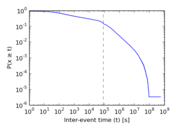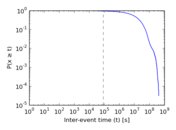### Matrix decompositions plots